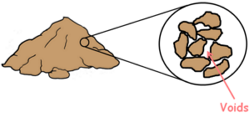Any soil when depositing contains some voids in them. The property of the soil which permits the water or any liquid to flow through it through its voids is called permeability. Permeability is the ease with which water can flow through the soils.

There are numerous methods through which we can measure the permeability of a soil in the field or of a representative sample in the laboratory.

In the laboratory we employ two methods

One is constant head permeability test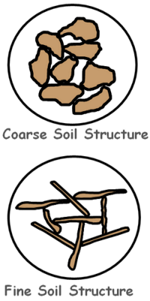These tests measure the amount of water that goes through a soil sample in a fixed time interval.

Constant head method is suitable for large grained soils which are relatively more pervious because of their larger voids. While variable head is more suitable for small grained soils which are relatively less pervious because of their poorly connected void structure.

We perform this test for fine grained soils where the quantity of discharge of liquid through them is very small.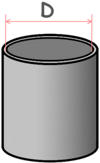For this test we use an instrument which consists of a cylindrical mould. We measure its internal diameter and determine its internal area which we say capital A.

This mould is attached with two filter discs at the top and the bottom. These discs are porous and highly permeable. These are also provided with inlet and outlet facilities.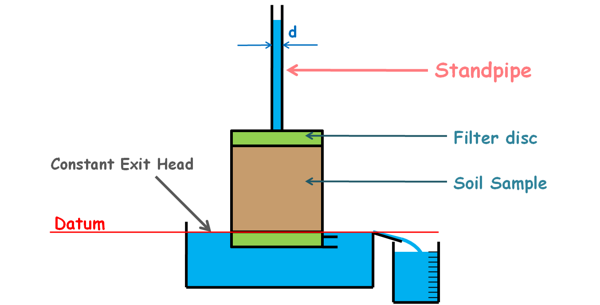Inlet is fitted with a graduated vertical standpipe. The diameter of this pipe is known and let’s say it is small letter d, also its area is small a.

We can perform this experiment on both disturbed and undisturbed soil samples.

We fill the mould with our sample soil and compact it to a density which may be representative of the field conditions.

Or for an undisturbed sample we take out the soil sample from the ground and trim it to the dimensions similar to that of the mould. We place this sample inside the mould between porous discs. Some space will be left between the mould and the specimen that we will fill with some impervious material such as cement slurry.

We fill the standpipe with water and before beginning the experiment we allow the water to flow through the soil and let it get fully saturated. Even after that we let the water to flow out for some time so that a steady flow can be attained.

A steady flow is the one in which the quantity of liquid flowing per second through any section, is constant.Then we close the knob at the outlet and stop the water flow. We refill the standpipe up to a certain height.

The height of the water column in the standpipe represents the head or the energy of the water. We assume there is negligible head loss due to the porous disc and the energy of the liquid starts dropping when it begins to flow through the soil. So if there is a manometer at the beginning of the soil its water level should be the same as that of in the pipe, because they are displaying the same water head. Hence we can think this inlet pipe as the head measurer at the beginning of the flow and we can ignore the first manometer.

Now if we have a manometer at the bottom of the sample and when water flows through the soil, the water level in the manometer will display the exit head of the water.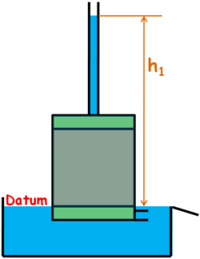But if we place the instrument in a tub like container full of water, its exit head will become constant. Also if we consider the exit head or water level of the container as datum then datum head is zero so exit head is also zero and initial inlet head of the water in the standpipe from datum is, say h1.

Hydraulic gradient, which is the difference of inlet head and outlet divided by the length of the soil specimen in which the flow takes place, will only depend on the height of the water column in the standpipe as outlet head is zero.When we open the outlet knob water comes out and overflow the container. We start a stopwatch and place a graduated jar to collect that overflow water.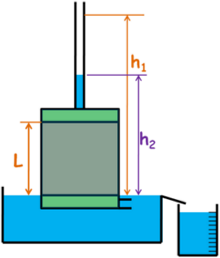We collect the water for a fixed time internal t and note down the amount of water collected as capital Q. We close the knob and also note down the final head in the standpipe which is also the final head difference from the datum. We call it h2.

Keep in mind that h1 and h2 are the heights of the water column in the standpipe and also the head differences which are causing the flow.

Now for this system we may apply Darcy‘s law which state that the velocity of flow of liquid between two points in the soil is directly proportional to the hydraulic gradient applied to it.

v α i

and K is added as proportionality constant which is called coefficient of permeability of soil.

V = ki

We also write the law for discharge rate as this.

q = kAi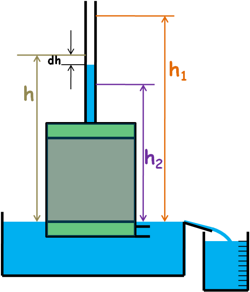We can notice the hydraulic gradient through the soil is changing every instant as the water head is continuously changing in the standpipe.

So let at any instant of time when the water head was in between h1 and h2, the head difference which was causing the flow through the soil is h. For a very short interval of time dt head in the standpipe drops by dh height. At that instant when the head is h the flow rate can be given as this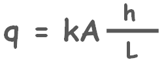and the amount of water collected in the jar with this rate in small time interval dt will be q.dt.

So using the continuity equation we can write volume of water dropped or decreased, negative sign for the decrease, in the standpipe is equal to the discharge of water collected or increased in the jar through the outlet.Volume of water dropped in the standpipe is area of standpipe multiplied by height of drop of water (a.dh) and the amount of water collected in the jar is discharge rate multiplied by time interval (q.dt).

-a.dh = q.dt

We can write discharge rate by Darcy’s law as this.

-a.dh = kAi.dt

Hydraulic gradient i for that instant of time was h/L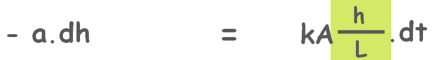We rearrange it and write it as this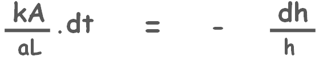Here some values are constant and for our taken test conditions permeability is constant. Only variables here are head difference and time. So we can integrate both from the head difference at initial head difference of h1 at time t equal to zero to the final head difference of h2 at time t equal to t.Now we can integrate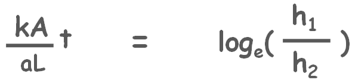and apply the limits also rearrange to find the value of permeability as this.Here log has its base e that can also be written as this for base 10.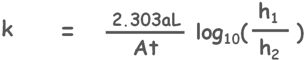In this equation we have measured and recorded all the required values to calculate and know the permeability of the soil sample.

According to Indian Standards we report the permeability values at 27 degree Celsius temperature. So if we measure the temperature of the water as T degree Celsius then using this formula we can calculate the permeability at 27 degree Celsius.Here K27 and KT are the permeability of the soil at 27 degree Celsius and at test temperature T degree Celsius respectively

myu 27 and myu T are the coefficient of viscosity at temperature 27 degree Celsius and test temperature T degree Celsius respectively

Tags : permeability

Published on :2020-03-25